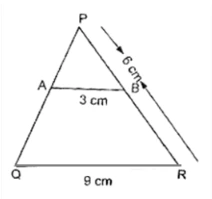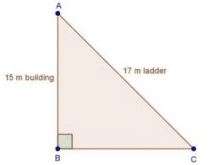# RD Sharma Class 10 and Chapter Triangles Maths Solutions

## Maths Class 10 and Chapter Triangles RD Sharma SolutionsFilter
Filters :
CLASSES
•
•
•
•
•
•
•
CHAPTERS
•
• 6 More

### RD SHARMA Class 10 | TRIANGLES

If A be the area of a right triangle and b one of the sides containing the right angle, prove that the length of the altitude on the hypotenuse is (2A B)/(sqrt(b^4+4A^2))### RD SHARMA Class 10 | TRIANGLES

Prove that the line segments joining the mid-points of the sides of a triangle from four triangles, each of which is similar to the original triangle.### RD SHARMA Class 10 | TRIANGLES

If a perpendicular is drawn from the vertex containing the right angle of a right triangle to the hypotenuse then prove that the triangle on each side of the perpendicular are similar to each other and to the original triangle. Also, prove that the square of the perpendicular is equal to the product of the lengths of the two parts of the hypotenuse.In this chapter, we will get some good understanding of Triangles. We will study different topics like similarity of Triangles, similar figures, areas of Triangle and criteria for similarity of Triangles. Doubtnut has provided solutions to exercises on the particular topic that proves to be of much use to the students in getting their queries solved. It also helps to attain the perfect understanding as to how it is possible to come up with the ideal solutions that also never take much time to solve it in the best manner.  So, it really helps a lot to achieve success in the exams.

The RD Sharma textbooks have been a reliable choice among the students studying under the CBSE syllabus. It is due to the clarity with which the concepts are put out and the depth in which the details are explained that the RD Sharma textbooks stand out from the rest of the books of this category. NCERT textbooks help students to clear the basic concepts, but when it comes to appearing for the papers, it proves to be quite in attempting the questions. In this case, RD Sharma books make it possible to clear all their queries where it helps students to get some good idea on how to solve different questions without taking a lot of time as well. In order to make things less complicated, the RD Sharma books contain numerous solved examples and practice exercises, but still students are not able to score good marks in the exams. There are times when the students remain uncertain of the method and approach that they follow to solve the problems. It is where a premium online tutorial platform like doubtnut.com comes to the rescue of the students. Students can find the self-explanatory video solutions of all the problems from RD Sharma’s books worked-out exercise-wise.

In order to help students in scoring higher marks, a team of Doubtnut has introduced the concept of online study materials where students can access the different chapters and topics in the best way. It can also be downloaded in the form of pdf. So, this truly helps to get an accurate idea on how to work on the different questions without any worry at all. Being flexible, it also helps a lot to work on the different exercises that make student gain good confidence out of it.

## Topics and subtopics of RD Sharma class 10 Chapter 4, Triangles

### Introduction

We have learned about triangles and also its properties. Here we will have some look at the exercises along with its solutions. So, let’s have a look at some of the important exercises:

Q.1)  Answer the following using the correct word

(1.) All circles are ____________ (congruent, similar)

(2.) All squares are ___________ (similar, congruent)

(3.) All ____________ triangles are similar (isosceles, equilaterals)

(4.) Two polygons of the same number of sides are similar, if (a) ____________their corresponding angles are and (b) ____________ their corresponding sides are (equal, proportional)

(5.) Two triangles are similar, if their corresponding sides are ____________ (proportional, equal)

Q.2)  Write the truth value (T/F) of each of the following statements:

(1.) Any two similar figures are congruent.

(2.) Any two congruent figures are similar.

(3.) Two polygons are similar if their sides are proportional.

(4.) Two polygons are similar if their corresponding sides are proportional.

(5.) Two triangles are similar if their corresponding angles are proportional.

(6.) Two triangles are similar if their corresponding sides are proportional.

Solution1:

(1)  Similar

(2)  Similar

(3)  Equilateral

(4) a) Equal, b) Proportional.

(5)  Proportional

Solution.2:

(1)   False

(2)  True

(3)  False

(4)  False

(5)  True

(6)  True

### Similar Figures

In order to get the right understanding about it, we will provide you with a solved exercise. This can help to clear your queries.

Exercise: In a Δ ABC, the points on the sides AB and AC are D and E respectively such that DE BC.

(i) If AD = 4 cm, AE = 8 cm, DB = x – 4 cm and EC = 3x – 19, find x.

Solution: Given: AD = 4 cm, AE = 8 cm, DB = x – 4 and EC = 3x – 19

To find x,

Hence, Using Thales Theorem,

Then, 4x–4 = 83x–19

4(3x – 19) = 8(x – 4)

12x – 76 = 8(x – 4)

12x – 8x = – 32 + 76

4x = 44 cm

x = 11 cm

(ii) If AD = x cm, DB = x – 2 cm, AE = x + 2 cm, and EC = x – 1 cm, find the value of x.

Solution:

It is given that AD = x, DB = x – 2, AE = x + 2 and EC = x – 1

We have to find the value of x

So, AD = AE ( using Thales Theorem)

BD    CE

Then.  x = x + 2

x-2   x  - 1

X (x-1) = (x-2)(x+2)

x2-x – x2 +4=0

x = 4

### Similarity of Triangles

It is important to note that triangle is also a polygon. Here, we will have a look at an exercise.

Exercise:  ΔACBΔAPQ If BC = 12 cm, PQ = 4 cm, BA = 6.5 cm, and AP = 2.8 cm find CA and AQ.

Solution:

Given,

ΔACBΔAPQ

BC = 12 cm, PQ = 4 cm, BA = 6.5 cm, and AP = 2.8 cm

To find= CA and AQ

We know that,

ΔACBΔAPQ

BA= CA = BC

AQ   AP     PQ

Then, 6.5 = 12

AQ     4

AQ = 6.5 x 4

12

AQ = 2.16 cm

In the same way, CA = BC

AP    PQ

CA= 12

2.8   4

CA = 2.8 x 3

CA =8.4cm

Therefore, CA = 8.4 cm and AQ = 2.16 cm.

Exercise: Note the diagram ABQR. Can you find the length of PB?Given,

ABPB

QR = 9 cm, AB = 3 cm,  and PR = 6 cm

To find= PB

We know that, AB = PB

QR   PR

= 3 = PB

9     6

Therefore PB = 2cm

### Criteria for Similarity of Triangles

When the corresponding angles are equal, then the two triangles are similar where the corresponding sides are in the same ratio. Let’s look at an exercise to get some good idea about it.

Exercise: Diagonals AC and BD of a trapezium ABCD with AB || DC intersect each other at the point O. By making use of similarity criterion for two triangles, show that OA = OB

OC    OD

Solution:

Given: OC is the point of intersection of AC and BD in the trapezium ABCD with AB DC.

To prove OA = OB

OC    OD

We know that,

From Δ AOB and Δ COD

AOB = COD (VOA)

OAB = OCD  (alternate angles)

Then, ΔAOBΔCOD

OA = OB   (corresponding sides are proportional)

OC    OD

### Pythagoras Theorem

We will now have a look at the use of Pythagoras Theorem. Let’s concentrate on the exercise below:

Exercise: A ladder 17 m long reaches a window 15 m above the ground. Calculate the distance of the foot of the ladder from the building.

Solution:From Δ ABC, by Pythagoras theorem,

AB2 + BC2 =AC2

= 152 + BC2= 172

= 225 +BC2 = 172

= BC3 = 289 – 225

= BC2= 64

= BC = 8m

So, the distance of the foot of the ladder from building = 8m

## Significance of Doubtnut for the students

Doubtnut has made it possible for students to clear all their queries in no time and worry-free.  All the topics have been explained in the simplest manner for which they do not find any sort of problem in understanding it. Let’s find out the importance of Doubtnut for the students.

• Provides to the point explanation:  The study materials designed by the expert tutors of Doubtnut help students to provide to the point explanation. With the help of the best examples and explanations, students get hands-on experience on how to deal with different questions in the best manner. So, it can be possible to get the best information on the technique on how to come up with an accurate solution.
• Expect the best support: Students can also expect the best support from Doubtnut that helps a lot to get rid of any queries. So, it is crucial to ensure opting for the best video tutorials that would surely help to find the exact idea on how to achieve good marks in the exams. It also becomes possible to develop a liking towards the subject as well. Therefore, if you can make the right use of the study materials, it would surely be possible to crack the exams without any worry at all.
• Becomes easy to solve any questions: It is also possible to find it much easier to solve even the trickiest question without any problem at all. By saving both time and money, your parents would feel proud of the best marks that you score in the examination.  So, if students are serious about their studies, then Doubtnut’s online study materials are the best choice for them.